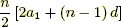## Week 17 in Precalculus 11

This week we started our final unit of precalc 11 which is trig. A total new concept on how to use it compared to grade 10.## Week 14 in Precalculus 11

This week we learned how to determine non-permissible values from rational expressions.In this question, you first start by taking out the denominator. With this denominator factor it to find the x values. The denominator can never equal zero. By finding finding the x’s you are finding the non-permissible values.

## Week 13 in Precalculus 11

This week I learned how to graph an absolute value of a quadratic function with a linear equation.Given the equation, start by plotting the y intercept which is +3. Then find the slop which isa rise of 3 and a run of 1. Once the graph hits the x-axis it reflects and continues the graph but with the opposites plots of when it would be below the x-axis.

## Week 12 in Precalculus 11

This week in Precalculus we learned how to solve systems of equations algebraically.Going step by step, substituting, simplifying and factoring to find the solutions for the equations.

## Week 11 in Precalculus 11

This week I learned how to graph a line and figure out what side has all possible solutions.

Example.With the line plot it on a graph by finding the y intercepts and the slope. Decide on if the line is solid or dotted by looking at the sign. if the sign is less than or greater than it is a dotted line, if it is less than or equal to, or greater than or equal to, then it is a solid line. By finding what side to shade, pick a plot from a side of the line and plug it into the equation. If the plots work with the equation then shade that side of the line where you got that point from, if it doesn’t shade the other side.

## Week 10 In Precalculus 11

This week in Precalculus was a review in preparation for our midterm. Three thing that I needed to review was solving radical expressions, converting standard to general form and our first chapter “Sequences and Series”.Above I have shown two questions one showing how to find the sum of a series, and one finding the the last term using the sum given in the question, by using the formulato find the sum and  an = a1 + (n – 1)d to find the nth term.

## Week 9 in Precalculus 11

Tell: Something we have learned this week is how to model and solve problems with quadratic functions.

Show:

One question I was stuck on was #5 on pg.323Explain:

I was stuck on this question because I didn’t know what to do after I got it into a=(20-w)w. Once I got help I discovered that I had to find the x intercepts and with that I proceeded to find my width, then plugged that into my equation I first made to find the length.

## Week 8 in Precalculus 11

Tell:

This week in Precalculus we learned how to analyze a graph to represent a quadratic function . For example finding the vertex, line of symmetry, and X – intercepts, Y – intercepts.

Show:Explain:

In the picture above I have shown a graph and labeled all the key information I have learned when analyzing a graph. Giving the definitions of each and then solving them.

## Week 7 in Precalculus 11Tell:

This week we learned how to find the root of the number by using the discriminant.

Show: